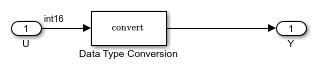## Detect Fixed-Point Constant Precision Loss

This example shows how to detect fixed-point constant precision loss.

### Open the Model

```open_system('ex_fixed_point_constant_precision_loss'); ```### Detect Fixed-Point Constant Precision Loss

For the Data Type Conversion block in this model:

• Input slope, SU = 1

• Output slope, SY = 1.000001

• Net slope, SU/SY = 1/1.000001

To set up the model and run the simulation:

1. For the Inport block, set the Data type to `int16`.

2. For the Data Type Conversion block, set the Output data type to `fixdt(1,16,1.000001,0)`.

3. In the Configuration Parameters dialog box, set the Diagnostics > Type Conversion > Detect precision loss configuration parameter to `error`.

4. In your Simulink® model window, in the Simulation tab, click Run.

When you simulate the model, a net slope quantization error occurs.

The Fixed-Point Designer™ software generates an error informing you that net scaling quantization caused precision loss. The message provides the following information:

• The block that introduced the error.

• The original value of the net slope.

• The quantized value of the net slope.

• The error in the value of the net slope.